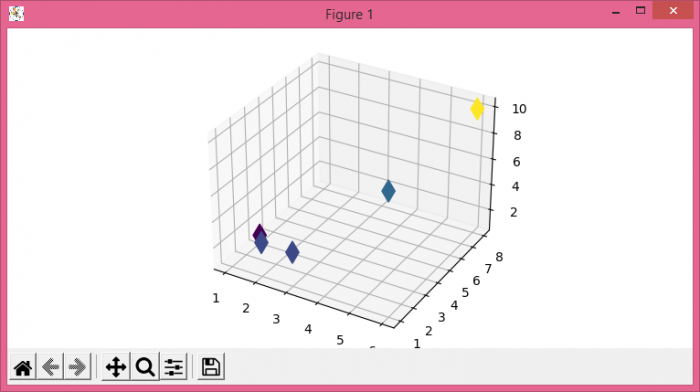# How to Zoom with Axes3D in Matplotlib?

To zoom with Axes3D, we can take the following steps −

• Set the figure size and adjust the padding between and around the subplots.
• Create a new figure or activate an existing figure using figure() method.
• Get 3D axes object using Axes3D(fig) method.
• Plot x, y and z data points using scatter() method.
• To display the figure, use show() method.

## Example

from mpl_toolkits.mplot3d import Axes3D
from matplotlib import pyplot as plt

plt.rcParams["figure.figsize"] = [7.50, 3.50]
plt.rcParams["figure.autolayout"] = True

fig = plt.figure()
ax = Axes3D(fig)
x = [2, 4, 6, 3, 1]
y = [1, 6, 8, 1, 3]
z = [3, 4, 10, 3, 1]

ax.scatter3D(x, y, z, c=z, alpha=1, marker='d', s=150)
plt.show()



## Output